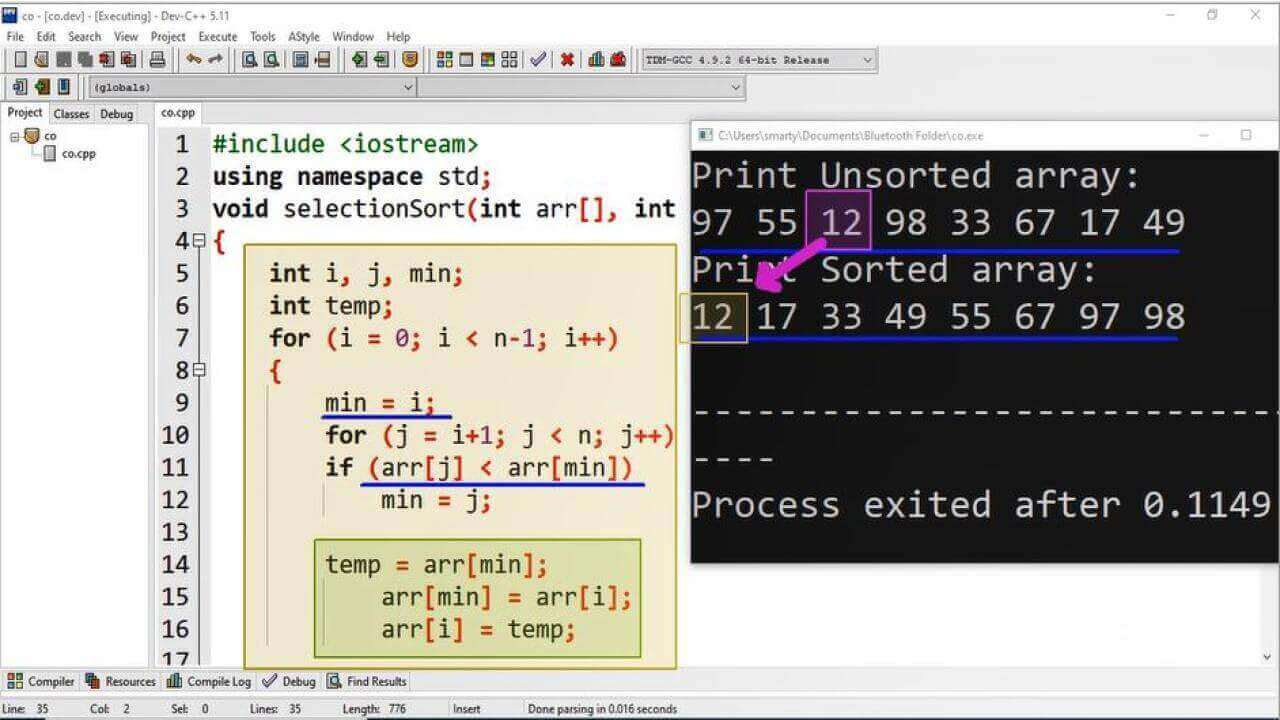# Selection Sort Program Using Cpp

## Source Code:

#include <iostream>
using namespace std;
void selectionSort(int arr[], int n)
// starting of function
int i, j, min // declaration of variables
int temp// temp variable for swapping values
for (i = 0; i < n-1; i++)  // outter loop
{
min = i// setting boundry of un-sorted array
for (j = i+1; j < n; j++)  // inner loop
if (arr[j] < arr[min])  // checking order of element
min = j;
// These lines of code will swap min value with next index of sorted array
temp = arr[min];
arr[min] = arr[i];
arr[i] = temp;
}   // ending of outter loop
// ending of function
int main()
{
int i// counter variable for printing array values
int arr[] = {97,55,12,98,33,67,17,49};  //array initialization
int n = sizeof(arr)/sizeof(arr);  // calculating no of elements
cout<<"Print Unsorted array: \n";
for (i = 0; i < n; i++) {  // printing array values before sort
cout << arr[i] << " ";

}
cout<<endl;
selectionSort(arr, n);  // function call for sorting
cout<<"Print Sorted array: \n";
for (i = 0; i < n; i++) {  // printing array values after sort
cout << arr[i] << " ";

}
cout << endl;
return 0;
}

## Output:## Working:

In this program example we have use a function named as selectionSort for implementation of algorithm. We have declare here four variables of int data type. The detail of these variables is provided as below.

 Sr. Variable Name / Identifier Data Type Description 1 i int This is counter variable used in outter loop. 2 j int This is counter variable used in inner loop. 3 min int This variable is used to track the boundry index of sorted and un-sorted array. 4 temp int Temp variable is used in swapping operation as a third variable.

After declaration process we have used nested loop for complete sorting process. The outter loop will set first counter variable value as 0 and go until N-1. In inner loop the entire logic is implemented we have set minimum index as the i and then traverse array from i+1 to N to find out if any element is also smaller than the already set minimum index.

Here in if statement we have used  <  symbol because we are going to sort array in Ascending order. Alternatively we have to use  symbol if we want to sort data in Descending order.

Finally in main() function we have initialized an array with 8 elements and also declare a variable as counter variable. In main function we print array elements before and after sorting function call. We have first print array elements and then call selectionSort function and after sorting we again print elements of array.